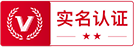## OneFlow如何做静态图的算子对齐任务

### 1、前言

OneFlow 提供了 nn.Graph 模块，让用户可以用类似 Eager 模式的编程习惯，构建静态图训练测试。因此，需要同时保证 Eager 和 Graph 模式下算子行为和结果的正确性。

https://github.com/Oneflow-Inc/oneflow/blob/master/python/oneflow/test_utils/automated_test_util/torch_flow_dual_object.py

https://github.com/Oneflow-Inc/oneflow/blob/master/python/oneflow/test_utils/automated_test_util/generators.py

### 2、OneFlow 的 Graph 算子对齐概述

OneFlow 提供的 Eager 模式，用法与 PyTorch 对齐。所以在测试上，AutoTest 框架会随机出各种合法参数组合成的 Op ，并基于数值和类型完全相同的输入 Tensor（PyTorch 和 OneFlow 各有一份）分别运行 PyTorch 和 OneFlow 的代码，来完成算子对齐工作。

`@autotest(check_graph = True) def test_flow_matmul_with_random_data(test_case): device = random_device() k = random(1, 6) x = random_tensor(ndim=2, dim1=k).to(device) y = random_tensor(ndim=2, dim0=k).to(device) z = torch.matmul(x, y) return z`

### 3.1 AutoTest 流程介绍

`GetDualObject`函数会重写传入的原始 `PyTorch`以及 `OneFlow`对象的 `__call__`魔法函数，最后返回一个 `DualObject`对象。这个过程中还包含跳过一些不需要关注的魔法函数，检查传入对象的属性是否合法，基于 `nn.Module`和其它 API 默认参数的类型对 `generator`继承类产生的随机数据绑定特定类型的工作（ `get_args`函数中完成）。此外，在代码中还有对 `tensor`方法的特判，因为 `tensor`方法的调用方式（通过 `getattr`）和 `nn.module`、 `nn.functional`不同（通过 `__call__`）。

`GetDualObject`函数实现：https://github.com/Oneflow-Inc/oneflow/blob/7fe29cb3d24be41fa981c4ad6be3051dacc3b605/python/oneflow/test_utils/automated_test_util/torch_flow_dual_object.py#L600

`DualObject`类对象实现在：https://github.com/Oneflow-Inc/oneflow/blob/0826518cc49200dccada0f54d5c83accb9218c83/python/oneflow/test_utils/automated_test_util/torch_flow_dual_object.py#L784

### 3.2 Graph 模式如何伴随 Eager 模式做算子对齐

`# NOTE(lixiang): When oneflow is of type nn.Module, build the following Graph for testing.# graph_train_oneflow: is a deepcopy of oneflow.def get_module_graph_test(graph_train_oneflow, oneflow, *args): of_sgd = flow.optim.SGD(graph_train_oneflow.parameters(), lr=0.001, momentum=0.9,) graph_train_parameters_len = 0 for param in oneflow._parameters.values(): if param is not None: graph_train_parameters_len += 1 class TestGraphOfModule(flow.nn.Graph): def __init__(self): super().__init__() self.test_module = graph_train_oneflow if global_backward and graph_train_parameters_len: self.add_optimizer(of_sgd) def build(self, *args): res = self.test_module(*args) forward_res = res if global_backward and graph_train_parameters_len: res = res.sum() res.backward() return forward_res return TestGraphOfModule()`

`# NOTE(lixiang): Check if the results of eager and graph are equal when oneflow is of type nn.Module or functional.def oneflow_eager_run_with_graph_check( oneflow, oneflow_args, oneflow_kwargs, testing_graph, verbose, *args): if testing_graph: graph_args, graph_kwargs = get_args_copy(oneflow_args, oneflow_kwargs) if isinstance(oneflow, flow.nn.Module): graph_train_oneflow = copy.deepcopy(oneflow) if not is_global(): arg_device_type = "cpu" for arg in oneflow_args: if flow.is_tensor(arg): arg_device_type = arg.device.type graph_train_oneflow = graph_train_oneflow.to(arg_device_type) else: graph_functional_oneflow = copy.deepcopy(oneflow) oneflow_res = get_oneflow_eager_res(oneflow, oneflow_args, oneflow_kwargs, verbose) if testing_graph: if verbose: print( "After running eager module or functional: ", repr(oneflow), ) find_check_module_func = True ignore_apis_list = ["tensor", "train"] test_g_res = [] if isinstance(oneflow, flow.nn.Module): test_g = get_module_graph_test(graph_train_oneflow, oneflow, *args) if verbose: print("Run graph of module: ", repr(oneflow)) test_g.debug(3) # When testing module methods, kwargs are not considered. test_g_res = test_g(*graph_args) if verbose: print( "The result after running graph module: ", test_g_res, ) elif oneflow.__name__ in ignore_apis_list: find_check_module_func = False # 1. "oneflow.nn.modules" not in oneflow.__module__: For avoid run nn.Module branch graph test, like fold op call Fold Module actually. # 2. inspect.isfunction(oneflow): Compared with the ordinary flow.xxx, oneflow.nn.modules.math_ops series op exist an extra layer of python wrapper. # 3. inspect.ismethod(oneflow) and "oneflow.nn.modules" in oneflow.__module__: For op that only has Tensor.xxx method, and call oneflow.xxx actually, like masked_fill. elif ( ("oneflow.nn.modules" not in oneflow.__module__) or inspect.isfunction(oneflow) or ( inspect.ismethod(oneflow) and "oneflow.nn.modules" in oneflow.__module__ ) ): test_g_res = get_functional_graph_res( graph_functional_oneflow, oneflow, oneflow_res, oneflow_args, oneflow_kwargs, verbose, *graph_args, **graph_kwargs, ) if find_check_module_func: if isinstance(test_g_res, tuple): for _, g_res in enumerate(test_g_res): check_eager_graph_tensor(oneflow_res, g_res) else: check_eager_graph_tensor(oneflow_res, test_g_res) return oneflow_res`

`if testing_graph: ··· ··· if isinstance(oneflow, flow.nn.Module): ··· test_g = get_module_graph_test(graph_train_oneflow, oneflow, *args) ··· elif: ··· ···`

### 3.3 Graph 模式的自动测试个性化

`if ( global_backward and graph_train_parameters_len): self.add_optimizer(of_sgd)·········if ( global_backward and graph_train_parameters_len): res = res.sum() res.backward()`

`# NOTE(lixiang): Deepcopy the input parameters in order to correctly test the inplace version of the op.def get_args_copy(args, kwargs): copy_args = [] for arg in args: if flow.is_tensor(arg): copy_arg = arg.clone().detach() else: copy_arg = copy.deepcopy(arg) copy_args.append(copy_arg) copy_kwargs = {} for key, value in kwargs.items(): if flow.is_tensor(value): copy_kwargs[key] = value.clone().detach() else: copy_kwargs[key] = copy.deepcopy(value) return copy_args, copy_kwargs`

### 4、Graph 的 Debug 支持

Graph 模式支持了学习率的调试输出，开启方法和 Eager 相同。

`optimizer = flow.optim.SGD(model.parameters(), lr=1e-3)# Set verbose=Truescheduler = flow.optim.lr_scheduler.CosineDecayLR(optimizer, decay_steps=100, alpha=0.98, verbose=True)`

`graph.debug(v_level = 1) # 可以简写为：graph.debug(1)`

`v_level=0`时，只输出最基础的警告和构图阶段信息，如构图时间。

`v_level=1`时，将额外打印每个 `nn.Module`的构图信息。

`v_level=2`时，在构图阶段，将额外打印每个 Op 的创建信息，包括名称、输入内容、设备和 SBP 信息等。

`v_level=3`时，将额外打印每个 Op 更详细的信息，如与代码位置有关的信息，方便定位代码问题。

### 5、总结

AutoTest 框架的灵活性和易用性都比较强，本文主要介绍了 Graph 模式如何伴随 Eager 模式做算子对齐和 Graph 的自动测试个性化内容。Eager 到 Graph 的 Local ops 执行测试覆盖也已经在 OneFlow v0.7.0中完成，在 0.8 版本中将会保证 Graph Global ops 单测的正确性。此外，静态图的 debug 和其他功能等将更完备。欢迎大家学习或者使用。

### 相关链接

1. https://github.com/Oneflow-Inc/oneflow

2. https://github.com/pytorch/pytorch

DeepMind爆发史：决定AI高峰的“游戏玩家”

LLVM之父Chris Lattner：编译器的黄金时代

OneFlow学习笔记：从Functor到OpExprInterpreter

OneFlow v0.7.0发布：全新分布式接口，LiBai、Serving等一应俱全

### 超前放送